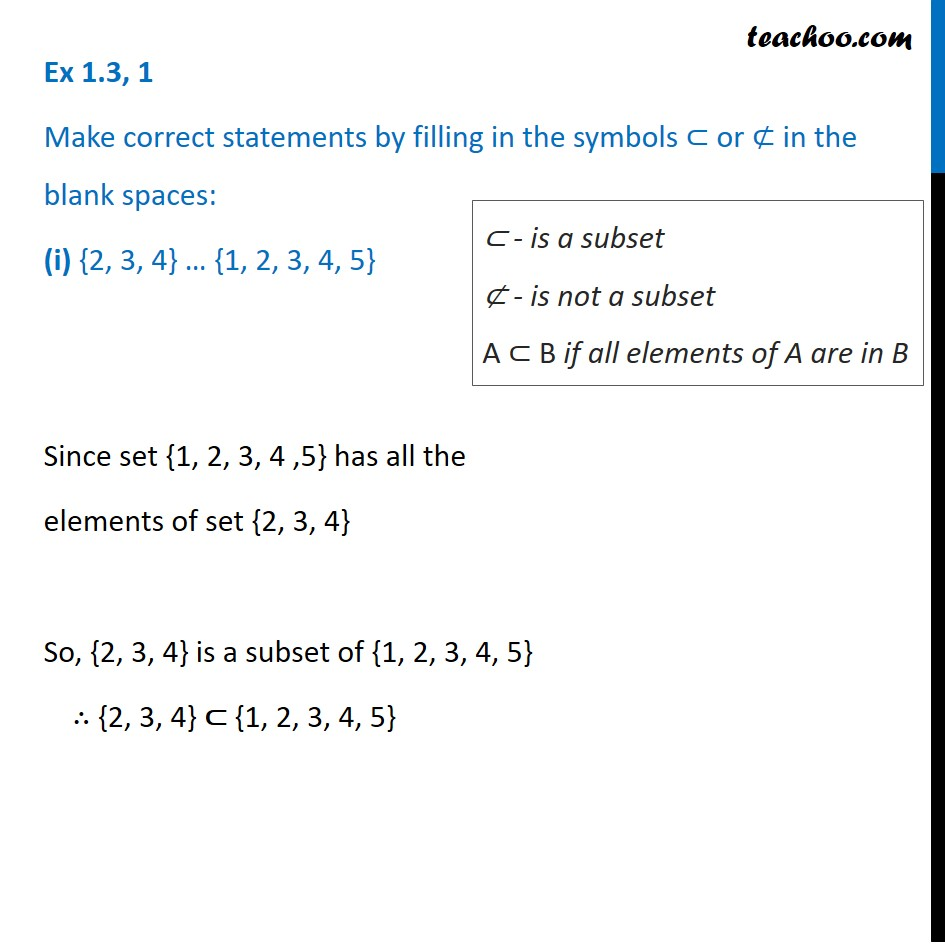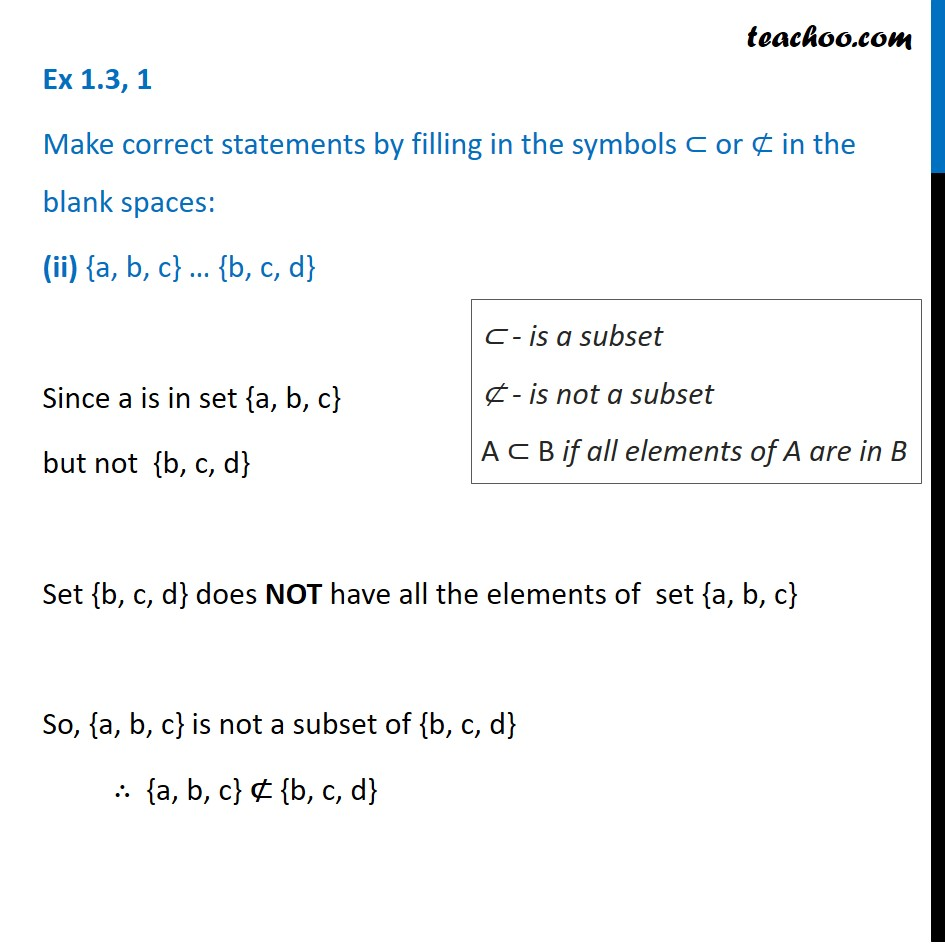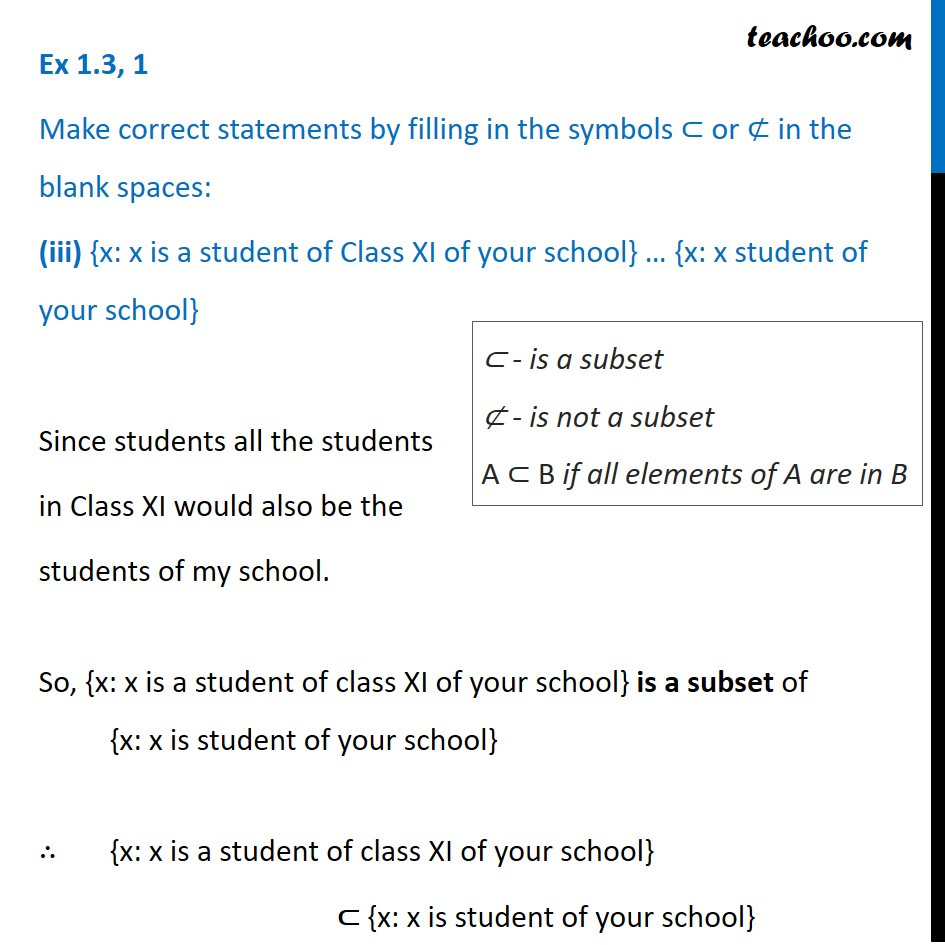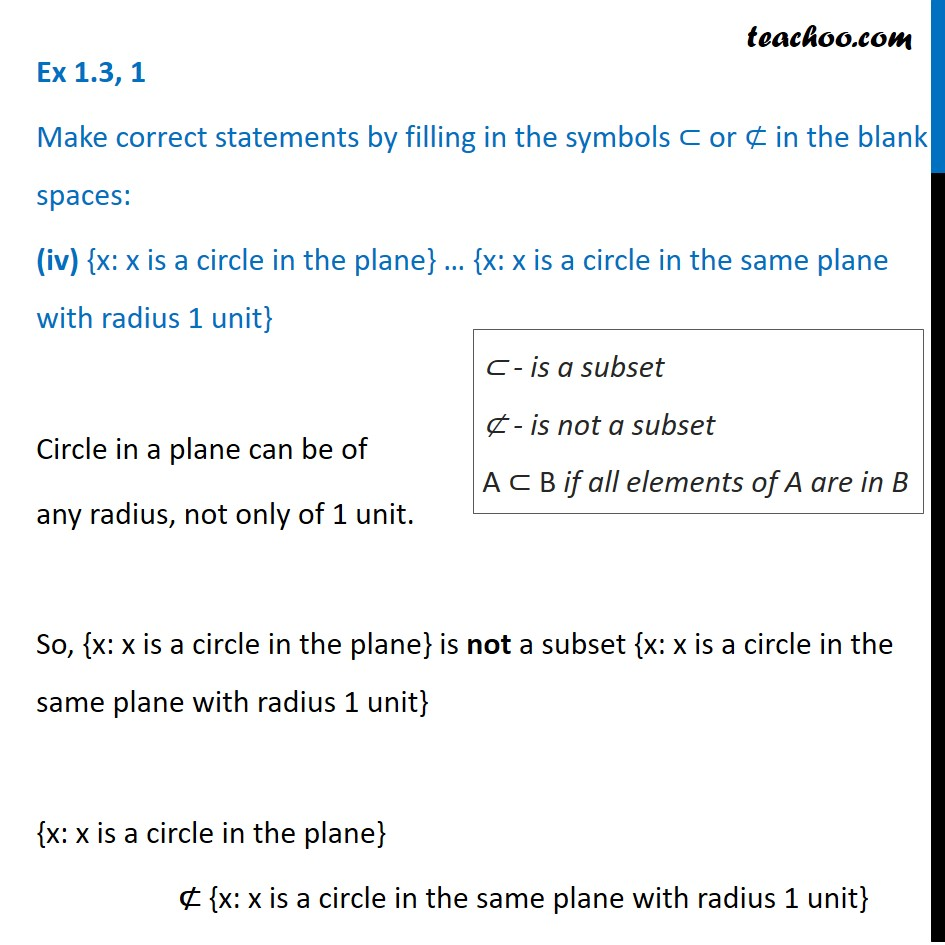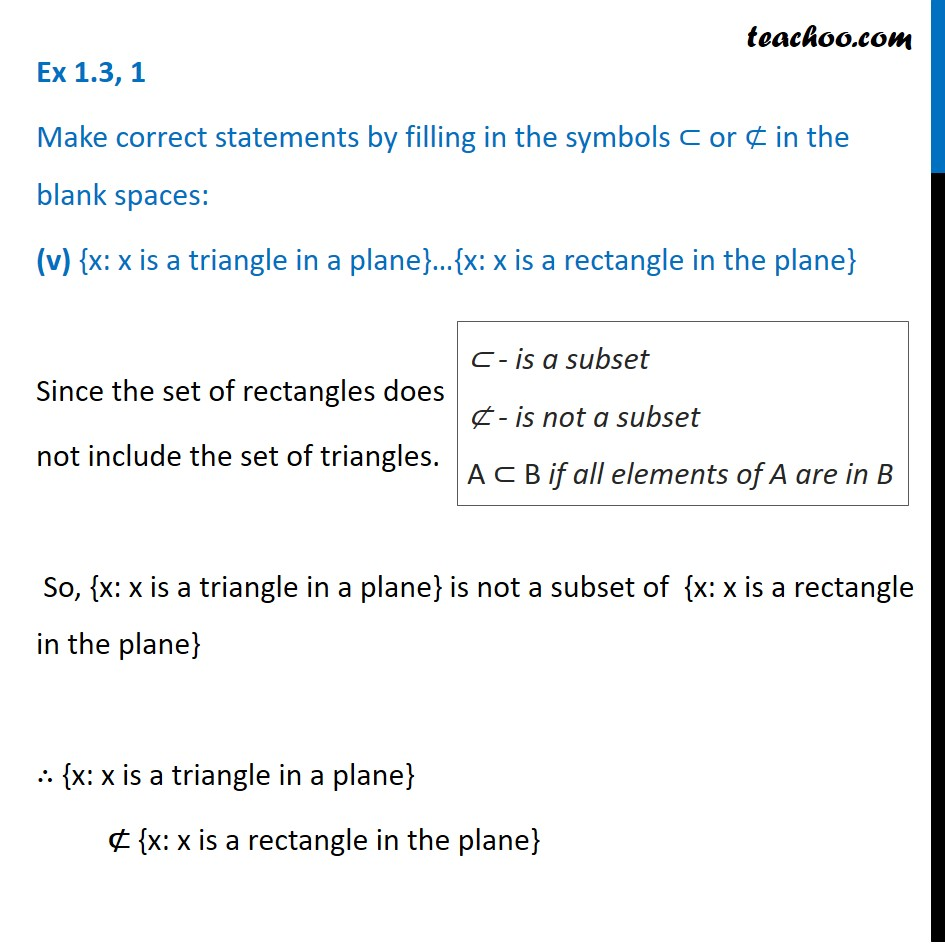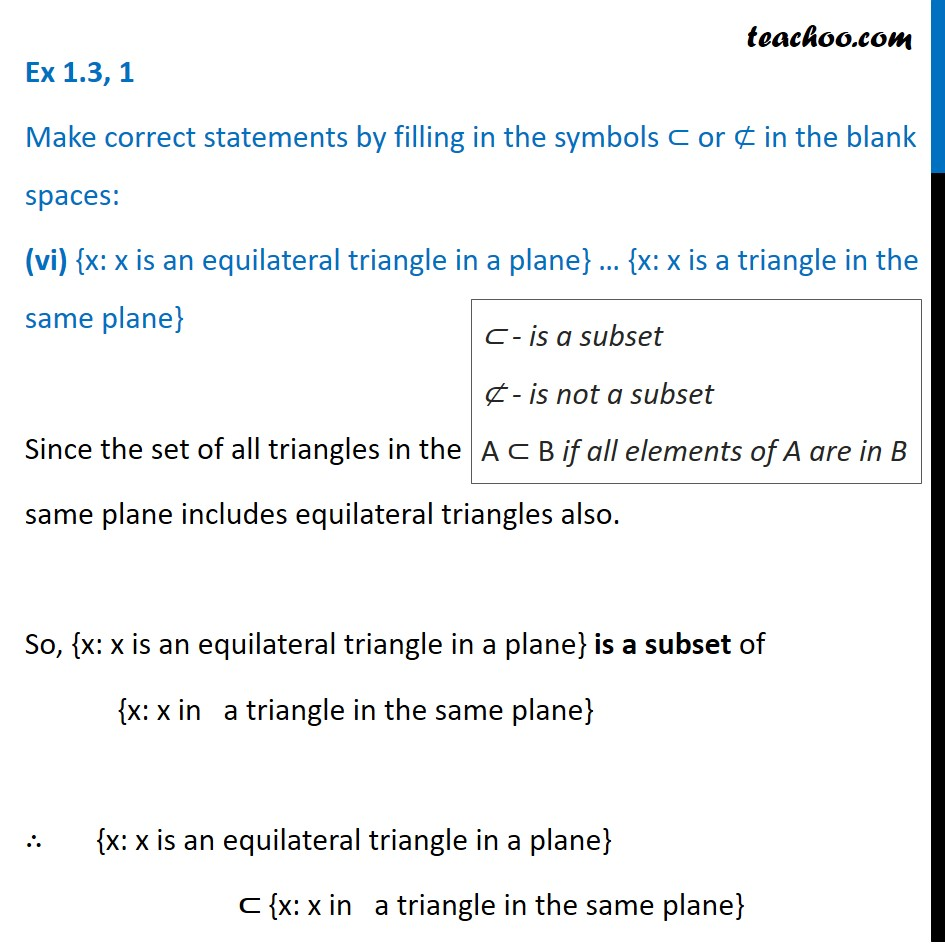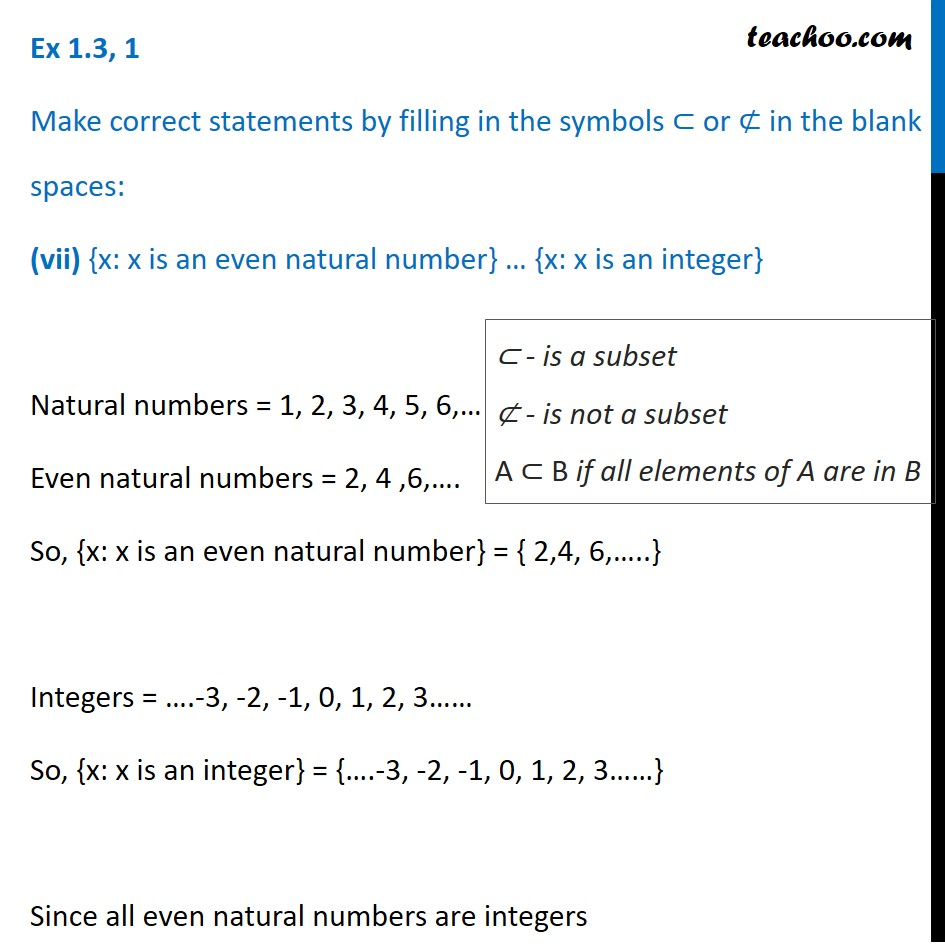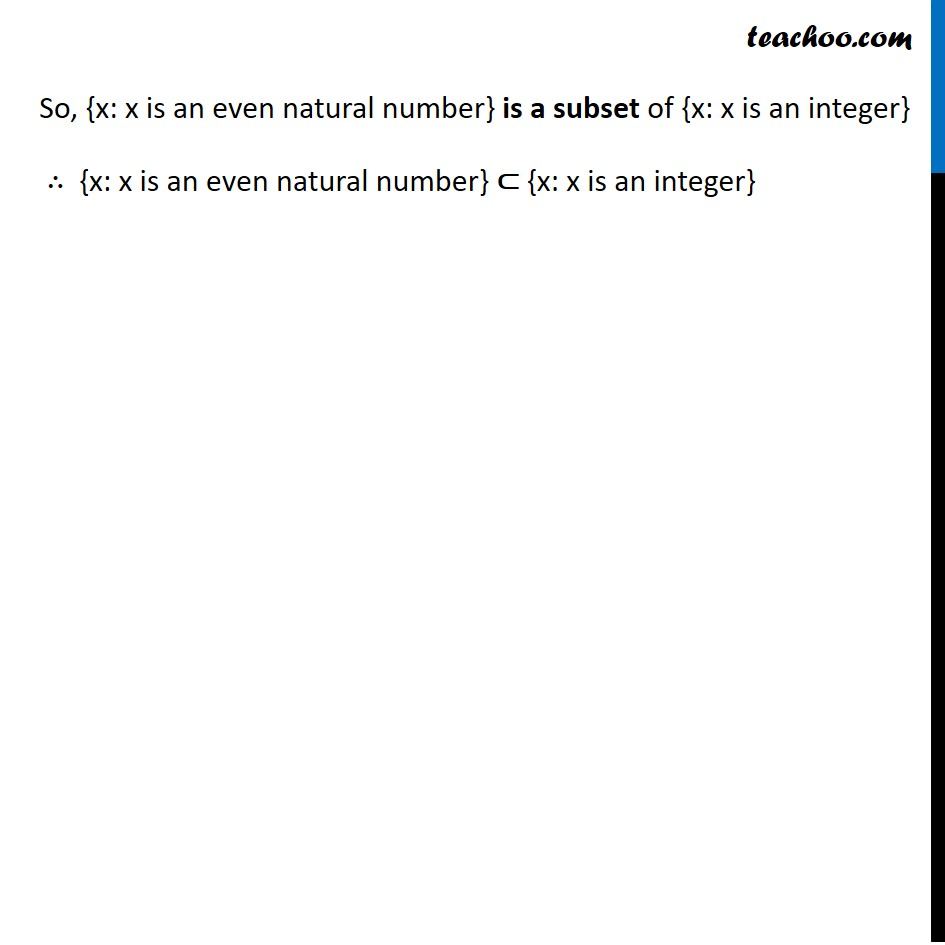1. Chapter 1 Class 11 Sets (Term 1)
2. Serial order wise
3. Ex 1.3

Transcript

Ex 1.3, 1 Make correct statements by filling in the symbols ⊂ or ⊄ in the blank spaces: (i) {2, 3, 4} … {1, 2, 3, 4, 5} Since set {1, 2, 3, 4 ,5} has all the elements of set {2, 3, 4} So, {2, 3, 4} is a subset of {1, 2, 3, 4, 5} ∴ {2, 3, 4} ⊂ {1, 2, 3, 4, 5} ⊂ - is a subset ⊄ - is not a subset A ⊂ B if all elements of A are in B Ex 1.3, 1 Make correct statements by filling in the symbols ⊂ or ⊄ in the blank spaces: (ii) {a, b, c} … {b, c, d} Since a is in set {a, b, c} but not {b, c, d} Set {b, c, d} does NOT have all the elements of set {a, b, c} So, {a, b, c} is not a subset of {b, c, d} ∴ {a, b, c} ⊄ {b, c, d} ⊂ - is a subset ⊄ - is not a subset A ⊂ B if all elements of A are in B Ex 1.3, 1 Make correct statements by filling in the symbols ⊂ or ⊄ in the blank spaces: (iii) {x: x is a student of Class XI of your school} … {x: x student of your school} Since students all the students in Class XI would also be the students of my school. So, {x: x is a student of class XI of your school} is a subset of {x: x is student of your school} ∴ {x: x is a student of class XI of your school} ⊂ {x: x is student of your school} ⊂ - is a subset ⊄ - is not a subset A ⊂ B if all elements of A are in B Ex 1.3, 1 Make correct statements by filling in the symbols ⊂ or ⊄ in the blank spaces: (iv) {x: x is a circle in the plane} … {x: x is a circle in the same plane with radius 1 unit} Circle in a plane can be of any radius, not only of 1 unit. So, {x: x is a circle in the plane} is not a subset {x: x is a circle in the same plane with radius 1 unit} {x: x is a circle in the plane} ⊄ {x: x is a circle in the same plane with radius 1 unit} ⊂ - is a subset ⊄ - is not a subset A ⊂ B if all elements of A are in B Ex 1.3, 1 Make correct statements by filling in the symbols ⊂ or ⊄ in the blank spaces: (v) {x: x is a triangle in a plane}…{x: x is a rectangle in the plane} Since the set of rectangles does not include the set of triangles. So, {x: x is a triangle in a plane} is not a subset of {x: x is a rectangle in the plane} ∴ {x: x is a triangle in a plane} ⊄ {x: x is a rectangle in the plane} ⊂ - is a subset ⊄ - is not a subset A ⊂ B if all elements of A are in B Ex 1.3, 1 Make correct statements by filling in the symbols ⊂ or ⊄ in the blank spaces: (vi) {x: x is an equilateral triangle in a plane} … {x: x is a triangle in the same plane} Since the set of all triangles in the same plane includes equilateral triangles also. So, {x: x is an equilateral triangle in a plane} is a subset of {x: x in a triangle in the same plane} ∴ {x: x is an equilateral triangle in a plane} ⊂ {x: x in a triangle in the same plane} ⊂ - is a subset ⊄ - is not a subset A ⊂ B if all elements of A are in B Ex 1.3, 1 Make correct statements by filling in the symbols ⊂ or ⊄ in the blank spaces: (vii) {x: x is an even natural number} … {x: x is an integer} Natural numbers = 1, 2, 3, 4, 5, 6,… Even natural numbers = 2, 4 ,6,…. So, {x: x is an even natural number} = { 2,4, 6,…..} Integers = ….-3, -2, -1, 0, 1, 2, 3…… So, {x: x is an integer} = {….-3, -2, -1, 0, 1, 2, 3……} Since all even natural numbers are integers ⊂ - is a subset ⊄ - is not a subset A ⊂ B if all elements of A are in B So, {x: x is an even natural number} is a subset of {x: x is an integer} ∴ {x: x is an even natural number} ⊂ {x: x is an integer}

Ex 1.3